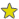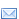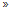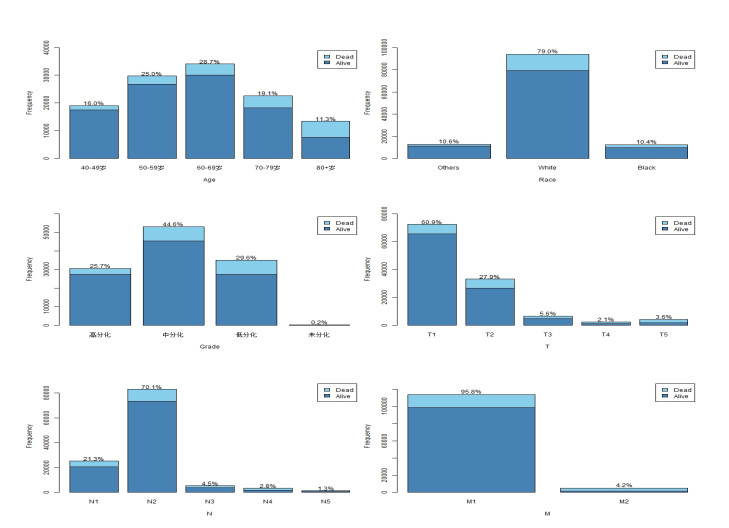﻿ 病例队列设计下加性风险回归分析及其在乳腺癌数据中的应用
数学杂志2021, Vol. 41Issue (3): 270-282PDF
 扩展功能加入收藏夹复制引文信息加入引用管理器Email AlertRSS 本文作者相关文章杨杰丁洁丽

ADDITIVE HAZARDS REGRESSION UNDER CASE-COHORT DESIGN AND ITS APPLICATION IN BREAST CANCER DATA
YANG Jie, DING Jie-liSchool of Mathematics and Statistics, Wuhan University, Wuhan 430072, China
Abstract: In this paper, we discuss how to fit the additive hazards model to case-cohort data. We consider a weighted estimating estimation approach, and review asymptotic properties of the proposed estimator. We conduct a series of simulation studies to assess the good finite-sample performance of such a method, and evaluate the efficiency of the case-cohort design to the simple random sampling scheme. We promote the traditional simple random sampling design to case-cohort design. We apply the proposed method to analyze a real data for breast cancer and illustrate the practical applications.
Keywords: case-cohort design     additive hazards model     estimating equations     inversed probability weighted
1 引言

2 加性风险模型与病例队列抽样

 \begin{align} \lambda(t{\mid}Z) = \lambda_{0}(t)+\beta^{'}Z_i(t), \end{align} (2.1)

 \begin{align} \widehat{\beta} = \left[\sum\limits_{i = 1}^{N}\int_{0}^{\tau}Y_{i}(t)\left\{Z_{i}(t)-\overline{Z}(t)\right\}^{\otimes2}dt\right]^{-1} \left[\sum\limits_{i = 1}^{N}\int_{0}^{\tau}\left\{Z_{i}(t)-\overline{Z}(t)\right\}dN_{i}(t)\right], \end{align} (2.3)

 \begin{align} \widehat{\Lambda}_{0}(t) = \int_{0}^{t}\frac{\sum\nolimits_{i = 1}^{N} \left\{dN_{i}(u)-Y_{i}(u)\widehat{\beta}^{\, '}Z_{i}(u)du\right\}}{\sum\nolimits_{i = 1}^{N}Y_{i}(u)}, \end{align} (2.4)

 $\overline{Z}(t) = \frac{\sum\nolimits_{i = 1}^{N}Y_{i}(t)Z_{i}(t)}{\sum\nolimits_{i = 1}^{N}Y_{i}(t)}.$

(C1) $\beta$的参数空间$\mathbb{B}$是紧集, $Z$的取值空间$\mathbb{Z}$是紧集.

(C2) 给定$Z_i$时, $\widetilde{T_i} $$C_i 相互独立, \xi_i$$ (T_i, \Delta_i, Z_i)$相互独立.

(C3) $P(T_{i}{\geq}\tau)>0 $$\Lambda_{0}(t)<\infty . (C4) 存在某个 \alpha \in (0, 1) , 使得当 N\rightarrow \infty 时, \widetilde{\alpha} = n_0/N \rightarrow \alpha . (C5) 矩阵  A = E\left[\int_{0}^{\tau}Y_{i}(t)\left\{Z_{i}(t)-\bar{z}(t)\right\}^{\otimes2}dt\right] 是非奇异矩阵, 其中  \bar{z}(t) = \frac{E\left[Y_i(t)Z_i(t)\right]}{E\left[Y_i(t)\right]}. 定理 1 ( \widehat{\beta}_w 的渐近性质) \label{thm1} 在正则条件(C1)-(C5) 下, \widehat{\beta}_w$$ \beta_0$的相合估计, 即:

 $\widehat{\beta}_w {\stackrel{\rm{P}}{\longrightarrow}} \beta_0.$

 $\sqrt{N}\{\widehat{\beta}_w-\beta_0\} {\stackrel{d}{\longrightarrow}} N(0, A^{-1}\left\{\Sigma_1+\Sigma_2\right\}{A^{-1}}),$

 $\Sigma_1 = E\left[\int_{0}^{\tau}\left\{Z_{i}(t)-\overline{z}(t)\right\}dM_{i}^{(0)}(t)\right]^{\otimes2},$
 $\Sigma_2 = \frac{1-\alpha}{\alpha} E\left[(1-\Delta_i)\int_{0}^{\tau}\left\{Z_{i}(t)-\overline{z}(t)\right\}dM_{i}^{(0)}(t)\right]^{\otimes2},$

 $M^{(0)}_i(t) = N_i(t)- \int_{0}^{t}Y_i(s)\left\{d\Lambda_0(s)+\beta_0^{'}Z_i(s)ds\right\}.$

 $\widehat{A} = \frac{1}{N} \sum\limits_{i = 1}^{N} \int_{0}^{\tau}Y_i(t)\left\{Z_i(t)- \overline{Z}_w(t)\right\}^{\otimes2}dt,$
 $\widehat{\Sigma}_1 = \frac{1}{N}\sum\limits_{i = 1}^{N}\omega_i\widehat{\varphi_i}^{\otimes2},$

 $\widehat{\Sigma}_2 = \left\{\frac{1-\widetilde{\alpha}}{\widetilde{\alpha}}\right\} \left[\frac{1}{N}\sum\limits_{i = 1}^{N}\omega_i\left(1-\Delta_i\right)\widehat{\varphi}_i^{\otimes2}\right],$

 $\widehat{\varphi}_i = \int_{0}^{\tau}\left\{Z_{i}(t)-\overline{Z}_w(t)\right\} \left\{dN_i(t)-Y_i(t)d\widehat{\Lambda}_0(t)-Y_i(t)\widehat{\beta}_w'Z_i(t)dt\right\}.$

 $\widehat{A}^{-1}\left\{\widehat{\Sigma}_1+\widehat{\Sigma}_2\right\}{\widehat{A}^{-1}}$

$A^{-1}\left\{\Sigma_1+\Sigma_2\right\}{A^{-1}}$的一个相合估计.

3 模拟研究

 $\lambda(t{\mid}Z) = \lambda_{0}(t)+\beta^{'}Z(t),$

Full: 基于全队列的估计方程方法($\widehat{\beta}_F$);

SRS: 基于子队列的估计方程方法($\widehat{\beta}_S$);

Naive: 基于与病例队列样本相同样本量的简单随机样本的估计方程方法($\widehat{\beta}_N$);

CC: 病例队列设计下的逆概率加权估计法($\widehat{\beta}_P$).表 1 参数β1β2的模拟结果, 其中Z1~ U(0, 1).表 2 参数β1β2的模拟结果, 其中Z1~ N(0, 1).

4 乳腺癌数据的生存分析图 1 影响因素条形图图 2 生存曲线条形图

 $\begin{equation*} \lambda(t{\mid}Z) = \lambda_0(t)+\beta_1 Age+\beta_2 Race+\beta_3 Grade+\beta_4 T+\beta_5 N+ \beta_6 M. \end{equation*}$表 3 乳腺癌细胞瘤研究数据分析结果

 $\frac{1}{N}\sum\limits_{i = 1}^{N}\omega_iY_{i}(t){\stackrel{\rm{a.s.}}{\longrightarrow}} E\left[Y_{i}(t)\right],$
 $\frac{1}{N}\sum\limits_{i = 1}^{N}\omega_iY_{i}(t)Z_{i}(t){\stackrel{\rm{a.s.}}{\longrightarrow}} E\left[Y_{i}(t)Z_{i}(t)\right].$

 \begin{align} \overline{Z}_w(t) {\stackrel{\rm{a.s.}}{\longrightarrow}} \bar{z}(t), \qquad \widehat{A} {\stackrel{\rm{a.s.}}{\longrightarrow}} A. \end{align} (A.1)

 \begin{align} \frac{1}{N}\sum\limits_{i = 1}^{N}\omega_i\int_{0}^{\tau}\left\{Z_i(t)-\overline{Z}_w(t)\right\}dN_i(t) = & \frac{1}{N}\sum\limits_{i = 1}^{N}\omega_i\int_{0}^{\tau}\left\{Z_{i}(t)-\overline{Z}_w(t)\right\}dM^{(0)}_i(t) \\ &+ \frac{1}{N}\sum\limits_{i = 1}^{N} \omega_i\int_{0}^{\tau}\left\{Z_{i}(t)-\overline{Z}_w(t)\right\}Y_{i}(t)d\Lambda_{0}(t) \\ &+ \left[ {\frac{1}{N} \sum\limits_{i = 1}^{N}\omega_i\int_{0}^{\tau}Y_i(t)\left\{Z_{i}(t)-\overline{Z}_w(t)\right\}^{\otimes2} Y_{i}(t)dt} \right]\beta_0 \\ {\stackrel{\rm{a.s.}}{\longrightarrow}}& A\beta_0. \end{align} (A.2)

 $\widehat{\beta}_w = \widehat{A}^{-1} \left[ {\frac{1}{N}\sum\limits_{i = 1}^{N}\omega_i\int_{0}^{\tau}\left\{Z_i(t)-\overline{Z}_w(t)\right\}dN_i(t)} \right] {\stackrel{\rm{a.s.}}{\longrightarrow}} \beta_0.$

 \begin{align} \sqrt{N}(\widehat{\beta}_w-\beta_0) = &\widehat{A}^{-1} \left[\frac{1}{\sqrt{N}}\sum\limits_{i = 1}^{N}\omega_i\int_{0}^{\tau} \left\{Z_{i}(t)-\overline{Z}_w(t)\right\}dM^{(0)}_{i}(t)\right] \\ = & \widehat{A}^{-1} \left[\frac{1}{\sqrt{N}}\sum\limits_{i = 1}^{N}\omega_i\int_{0}^{\tau}\left\{Z_{i}(t)-\bar{z}(t)\right\}dM^{(0)}_{i}(t)\right] \\ &- \widehat{A}^{-1}\left[\int_{0}^{\tau} \left\{\overline{Z}_w(t)-\bar{z}(t)\right\}d\left\{\frac{1}{\sqrt{N}}\sum\limits_{i = 1}^{N}\omega_idM_{i}^{(0)}(t)\right\}\right]. \end{align} (A.3)

 \begin{align} \sqrt{N}(\widehat{\beta}_w-\beta_0) & = A^{-1} \left[\frac{1}{\sqrt{N}}\sum\limits_{i = 1}^{N}\omega_i\int_{0}^{\tau}\left\{Z_{i}(t)-\bar{z}(t)\right\}dM^{(0)}_{i}(t) \right]+o_{P}(1) \\ & = A^{-1}\left[\frac{1}{\sqrt{N}}\sum\limits_{i = 1}^{N}\omega_i\varphi_i\right]+o_{P}(1). \end{align} (A.4)

 $\frac{1}{\sqrt{N}}\sum\limits_{i = 1}^{N}\omega_i\varphi_i = \frac{1}{\sqrt{N}}\sum\limits_{i = 1}^{N}\varphi_i+ \frac{1}{\sqrt{N}}\sum\limits_{i = 1}^{N}(\omega_i-1)\varphi_i.$

 $\begin{equation} \frac{1}{\sqrt{N}}\sum\limits_{i = 1}^{N}\varphi_i {\stackrel{d}{\longrightarrow}} N(0, \, \Sigma_1), \end{equation}$ (A.5)
 $\begin{equation} \frac{1}{\sqrt{N}}\sum\limits_{i = 1}^{N}(\omega_i-1)\varphi_i {\stackrel{d}{\longrightarrow}} N(0, \, \Sigma_2). \end{equation}$ (A.6)

 $\frac{1}{\sqrt{N}}\sum\limits_{i = 1}^{N}\omega_i\varphi_i {\stackrel{d}{\longrightarrow}} N(0, \, \Sigma_1+\Sigma_2).$

 $\sqrt{N}(\widehat{\beta}_w-\beta_0) {\stackrel{d}{\longrightarrow}} N(0, \, A^{-1}{(\Sigma_1+\Sigma_2)}A^{-1}).$

  Cox D R. Regression models and life-tables[J]. Journal of the Royal Statistical Society (SeriesB), Methodological, 1972, 34(2): 187–220.  Andersen P K, Gill R D. Cox's regression model for counting processes: a large sample study[J]. The Annals of Statistics, 1982, 10(4): 1100–1120.  Lin D Y, Ying Z. Semiparametric analysis of the additive risk model[J]. Biometrika, 1994, 81(1): 61–71. DOI:10.1093/biomet/81.1.61  Chen H Y, Little R J. Proportional hazards regression with missing covariates[J]. Journal of the American Statistical Association, 1999, 94(447): 896–908. DOI:10.1080/01621459.1999.10474195  John P Klein. The statistical analysis of failure time data[J]. Technometrics, 2012, 24(3): 251–251.  Mckeague I W, Sasieni P D. A partly parametric additive risk model[J]. Biometrika, 1994, 81(3): 501–514. DOI:10.1093/biomet/81.3.501  Zeng Donglin, Cai Jianwen. Additive transformation models for clustered failure time data[J]. Lifetime Data Analysis, 2010, 16(3): 333–352. DOI:10.1007/s10985-009-9145-1  Prentice R L. A case-cohort design for epidemiologic cohort studies and disease prevention trials[J]. Biometrika, 1986, 73(1): 1–11. DOI:10.1093/biomet/73.1.1  Self S G, Prentice R. Asymptotic distribution theory and efficiency results for case-cohort studies[J]. The Annals of Statistics, 1988, 16(1): 64–81.  Chen K, Lo S. Case-cohort and case-control analysis with Cox's model[J]. Biometrika, 1999, 86(4): 755–764. DOI:10.1093/biomet/86.4.755  Lin D Y, Ying Z. Cox regression with incomplete covariate measurements[J]. Journal of the American Statistical Association, 2012, 88(424): 1341–1349.  Kulich M, Lin D Y. Improving the efficiency of relative-risk estimation in case-cohort studies[J]. Journal of the American Statistical Association, 2004, 99(467): 832–844. DOI:10.1198/016214504000000584  Kulich M, Lin D Y. Additive hazards regression for case-cohort studies[J]. Biometrika, 2000, 87(1): 15. DOI:10.1093/biomet/87.1.15  Sun J, Sun L Q, Flournoy N. Additive hazards model for competing risks analysis of the case-cohort design[J]. Communications in Statistics-Theory and Methods, 2004, 33(2): 351–366. DOI:10.1081/STA-120028378  Kong L, Cai J, Sen P K. Weighted estimating equations for semiparametric transformation models with censored data from a case-cohort design[J]. Biometrika, 91(2), 305-319.  Lu W, Tsiatis A A. Semiparametric transformation models for the case-cohort study[J]. Biometrika, 2006, 93(1): 8.  Kong L, Cai J. Case-cohort analysis with accelerated failure time model[J]. Biometrics., 2009, 65(1): 135–142. DOI:10.1111/j.1541-0420.2008.01055.x  Kang S, Cai J, Chambless L. Marginal additive hazards model for case-cohort studies with multiple disease outcomes: an application to the Atherosclerosis Risk in Communities (ARIC) study[J]. Biostatistics, 2013, 14(1): 28–41. DOI:10.1093/biostatistics/kxs025  Kim S, Zheng D, Cai J. Analysis of multiple survival events in generalized case-cohort designs[J]. Biometrics, 2018, 74(4): 1250–1260. DOI:10.1111/biom.12923  Maitra Poulami, Amorim Leila D A F, Cai Jianwen. Multiplicative rates model for recurrent events in case-cohort studies[J]. Lifetime data analysis., 2020, 26(1): 134–157. DOI:10.1007/s10985-019-09466-0  陈津利, 莫邦豪, 冯可立. 乳腺癌患者在自助组织中的情感支持: 一个探索性的研究[J]. The Hong Kong Journal of Social Work, 2003, 37(2): 173–190.  https://seer.cancer.,gov/data/  Horvitz D G, Thompson D J. A generalization of sampling without replacement from a finite universe[J]. Journal of the American Statistical Association., 1952, 47(260): 663–685. DOI:10.1080/01621459.1952.10483446  Zeger Scott L, Liang Kung-Yee. Longitudinal data analysis for discrete and continuous outcomes[J]. Biometrics., 1986, 42(1): 121–130. DOI:10.2307/2531248  Kalbfleisch J D, Lawless J F. Likelihood analysis of multi-state models for disease incidence and mortality[J]. Statistics in medicine., 1988, 7(1-2): 149–160. DOI:10.1002/sim.4780070116  Pollard D. Empirical processes: theory and applications[J]. NSF-CBMS Regional Conference Series in Probability and Statistics, 1990, 2(1): 1–86.  van der Vaart AW, Wellner JA. Weak convergence and empirical processes[M]. NewYork: Springer, 1996.  Kulich Michal, Lin D Y. Additive hazards regression with covariate measurement Error[J]. Journal of the American Statistical Association., 2012, 95(449): 238–248.